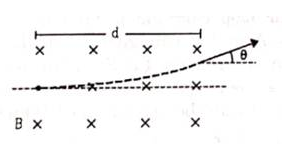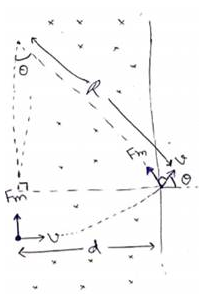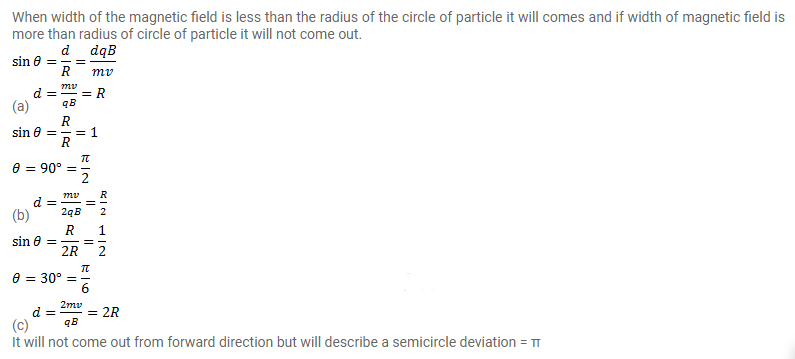# A particle of mass m and charge q is projected into a region having

Question:

A particle of mass $m$ and charge $q$ is projected into a region having a perpendicular magnetic field $B$. Find the angle of deviation of the particle as it comes out of the magnetic field if the width d of the region is very slightly smaller than

(a) $\frac{m v}{q B}$

(b) $\frac{m v}{2 q B}$

(c) $\frac{2 m v}{q B}$Solution: